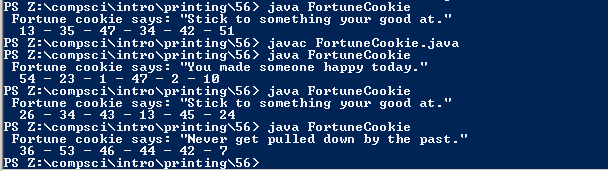# Assignemnt #56

## Code

```    ///Name: Joshua Bautista
///Period: 6
///Date: 1/7/2016

import java.util.Scanner;
import java.util.Random;

{
public static void main( String[]args )
{
Random r = new Random();
Scanner keyboard = new Scanner(System.in);
int num1, num2, num3, num4, num5, num6, fortune;

num1 = 1 + r.nextInt(54);
num2 = 1 + r.nextInt(54);
num3 = 1 + r.nextInt(54);
num4 = 1 + r.nextInt(54);
num5 = 1 + r.nextInt(54);
num6 = 1 + r.nextInt(54);
fortune = r.nextInt(5);

if ( fortune == 0 )
{
System.out.println("  " + num1 + " - " + num2 + " - " + num3 + " - " + num4 + " - " + num5 + " - " + num6 + "  " );
}
if ( fortune == 1 )
{
System.out.println(" Fortune cookie says: \"Sadness will come here and there but happiness will be forever.\"");
System.out.println("  " + num1 + " - " + num2 + " - " + num3 + " - " + num4 + " - " + num5 + " - " + num6 + "  " );
}
if ( fortune == 2 )
{
System.out.println("  " + num1 + " - " + num2 + " - " + num3 + " - " + num4 + " - " + num5 + " - " + num6 + "  " );
}
if ( fortune == 3 )
{
System.out.println("  " + num1 + " - " + num2 + " - " + num3 + " - " + num4 + " - " + num5 + " - " + num6 + "  " );
}
if ( fortune == 4 )
{
System.out.println(" Fortune cookie says: \"Never get pulled down by the past.\"");
System.out.println("  " + num1 + " - " + num2 + " - " + num3 + " - " + num4 + " - " + num5 + " - " + num6 + "  " );
if ( fortune == 5 )
{